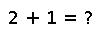# cat

cat(dims, A...)

Concatenate the input arrays along the specified dimensions in the iterable `dims`. For dimensions not in `dims`, all input arrays should have the same size, which will also be the size of the output array along that dimension. For dimensions in `dims`, the size of the output array is the sum of the sizes of the input arrays along that dimension. If `dims` is a single number, the different arrays are tightly stacked along that dimension. If `dims` is an iterable containing several dimensions, this allows to construct block diagonal matrices and their higher-dimensional analogues by simultaneously increasing several dimensions for every new input array and putting zero blocks elsewhere. For example, `cat([1,2], matrices...)` builds a block diagonal matrix, i.e. a block matrix with `matrices`, `matrices`, ... as diagonal blocks and matching zero blocks away from the diagonal.

## Examples

``````julia> foo = Array(Int8,2,2)
2x2 Array{Int8,2}:
2  0
0  0
julia> bar = Array(Int8,2,2)
2x2 Array{Int8,2}:
-93    -111
62             5
julia> cat(1,foo,bar)
4x2 Array{Int8,2}:
2      0
0      0
-93    -111
62     5
julia> cat(2,foo,bar)
2x4 Array{Int8,2}:
2      0       -93     -111
0      0       62      5``````

Checking you are not a robot: